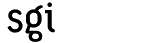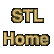# copy_nCategory: algorithms Component type: function

### Prototype

```template <class InputIterator, class Size, class OutputIterator>
OutputIterator copy_n(InputIterator first, Size count,
OutputIterator result);
```

### Description

Copy_n copies elements from the range [first, first + n) to the range [result, result + n). That is, it performs the assignments *result = *first, *(result + 1) = *(first + 1), and so on. Generally, for every integer i from 0 up to (but not including) n, copy_n performs the assignment *(result + i) = *(first + i). Assignments are performed in forward order, i.e. in order of increasing n. 

The return value is result + n.

### Definition

Defined in the standard header algorithm, and in the nonstandard backward-compatibility header algo.h. This function is an SGI extension; it is not part of the C++ standard.

### Requirements on types

• InputIterator is a model of Input Iterator.
• OutputIterator is a model of Output Iterator.
• Size is an integral type.
• InputIterator's value type is convertible to a type in OutputIterator's set of value types.

### Preconditions

• n >= 0.
• [first, first + n) is a valid range.
• result is not an iterator within the range [first, first + n).
• [result, result + n) is a valid range.

### Complexity

Linear. Exactly n assignments are performed.

### Example

```vector<int> V(5);
iota(V.begin(), V.end(), 1);

list<int> L(V.size());
copy_n(V.begin(), V.size(), L.begin());
assert(equal(V.begin(), V.end(), L.begin()));
```

### Notes

 Copy_n is almost, but not quite, redundant. If first is an input iterator, as opposed to a forward iterator, then the copy_n operation can't be expressed in terms of copy.